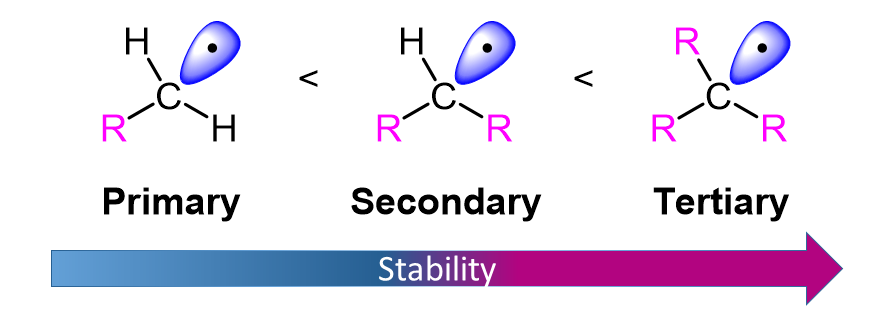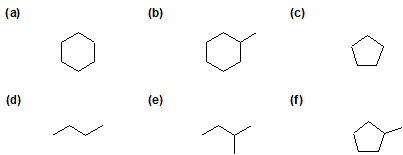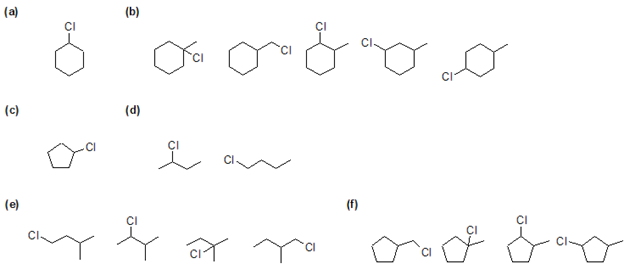# 10.2: Preparing Alkyl Halides from Alkanes - Radical Halogenation

$$\newcommand{\vecs}{\overset { \rightharpoonup} {\mathbf{#1}} }$$ $$\newcommand{\vecd}{\overset{-\!-\!\rightharpoonup}{\vphantom{a}\smash {#1}}}$$$$\newcommand{\id}{\mathrm{id}}$$ $$\newcommand{\Span}{\mathrm{span}}$$ $$\newcommand{\kernel}{\mathrm{null}\,}$$ $$\newcommand{\range}{\mathrm{range}\,}$$ $$\newcommand{\RealPart}{\mathrm{Re}}$$ $$\newcommand{\ImaginaryPart}{\mathrm{Im}}$$ $$\newcommand{\Argument}{\mathrm{Arg}}$$ $$\newcommand{\norm}{\| #1 \|}$$ $$\newcommand{\inner}{\langle #1, #2 \rangle}$$ $$\newcommand{\Span}{\mathrm{span}}$$ $$\newcommand{\id}{\mathrm{id}}$$ $$\newcommand{\Span}{\mathrm{span}}$$ $$\newcommand{\kernel}{\mathrm{null}\,}$$ $$\newcommand{\range}{\mathrm{range}\,}$$ $$\newcommand{\RealPart}{\mathrm{Re}}$$ $$\newcommand{\ImaginaryPart}{\mathrm{Im}}$$ $$\newcommand{\Argument}{\mathrm{Arg}}$$ $$\newcommand{\norm}{\| #1 \|}$$ $$\newcommand{\inner}{\langle #1, #2 \rangle}$$ $$\newcommand{\Span}{\mathrm{span}}$$

##### Objectives

After completing this section, you should be able to

1. explain why the radical halogenation of alkanes is not usually a particularly good method of preparing pure samples of alkyl halides.
2. use $$\ce{\sf{C–H}}$$ bond energies to account for the fact that in radical chlorinations, the reactivity of hydrogen atoms decreases in the order $\text{tertiary} > \text{secondary} > \text{primary}.$
3. predict the approximate ratio of the expected products from the monochlorination of a given alkane.
##### Study Notes

The following terms are synonymous:

1. methyl hydrogens, primary hydrogens, and 1° hydrogens.
2. methylene hydrogens, secondary hydrogens, and 2° hydrogens.
3. methine hydrogens, tertiary hydrogens, and 3° hydrogens.

Note that in radical chlorination reactions, the reactivity of methine, methylene and methyl hydrogens decreases in the ratio of approximately 5 : 3.5 : 1. This will aid in the prediction of expected products from the monochlorination of a given alkane.

Alkanes (the simplest of all organic compounds) undergo very few reactions. One of these reactions is halogenation, or the substitution of a single hydrogen on the alkane for a single halogen (Cl2 or Br2) to form a haloalkane. This reaction is very important in organic chemistry because it functionalizes alkanes which opens a gateway to further chemical reactions.

### General Reaction

$CH_4 + Cl_2 + energy → CH_3Cl + HCl$

The reaction proceeds through the radical chain mechanism which is characterized by three steps: initiation, propagation, and termination. Initiation requires an input of energy but after that the reaction is self-sustaining.

#### Step 1: Initiation

During the initiation step free radicals are created when ultraviolet light or heat causes the X-X halogen bond to undergo homolytic to create two halogen free radicals. It is important to note that this step is not energetically favorable and cannot occur without some external energy input. After this step, the reaction can occur continuously (as long as reactants provide) without input of more energy.#### Step 2: Propagation

The next two steps in the mechanism are called propagation steps. In the first propagation step, a chlorine radical abstracts hydrogen atom from methane. This gives hydrochloric acid (HCl, the inorganic product of this reaction) and the methyl radical. In the second propagation step, the methyl radical reacts with more of the chlorine starting material (Cl2). One of the chlorine atoms becomes a radical and the other combines with the methyl radical to form the alkyl halide product.#### Step 3: Termination

In the three termination steps of this mechanism, radicals produced in the mechanism an undergo radical coupling to form a sigma bond. These are called termination steps because a free radical is not produced as a product, which prevents the reaction from continuing. Combining the two types of radicals produced can be combined to from three possible products. Two chlorine radicals and couple to form more halogen reactant (Cl2). A chlorine radical and a methyl radical can couple to form more product (CH3Cl). An finally, two methyl radicals can couple to form a side product of ethane (CH3CH3).This reaction is a poor synthetic method due to the formation of polyhalogenated side products. The desired product occurs when one of the hydrogen atoms in the methane has been replaced by a chlorine atom. However, the reaction doesn't stop there, and all the hydrogens in the methane can in turn be replaced by chlorine atoms to produce a mixture of chloromethane, dichloromethane, trichloromethane and tetrachloromethane.## Energetics

Why do these reactions occur? Is the reaction favorable? A way to answer these questions is to look at the change in enthalpy ΔH that occurs when the reaction takes place.

ΔH = (Energy put into reaction) – (Energy given off from reaction)

If more energy is put into a reaction than is given off, the ΔH is positive, the reaction is endothermic and not energetically favorable. If more energy is given off in the reaction than was put in, the ΔH is negative, the reaction is said to be exothermic and is considered favorable. The figure below illustrates the difference between endothermic and exothermic reactions.ΔH can also be calculated using bond dissociation energies (ΔH°):

$\Delta{H} = \sum \Delta{H^°} \text{ of bonds broken} - \sum \Delta{H^°} \text{ of bonds formed}$

Let’s look at our specific example of the chlorination of methane to determine if it is endothermic or exothermic:Since, the ΔH for the chlorination of methane is negative, the reaction is exothermic. Energetically this reaction is favorable. In order to better understand this reaction we need to look at the mechanism ( a detailed step by step look at the reaction showing how it occurs) by which the reaction occurs.

## Chlorination of Other Alkanes

When alkanes larger than ethane are halogenated, isomeric products are formed. Thus chlorination of propane gives both 1-chloropropane and 2-chloropropane as mono-chlorinated products. The halogenation of propane discloses an interesting feature of these reactions. All the hydrogens in a complex alkane do not exhibit equal reactivity. For example, propane has eight hydrogens, six of them being structurally equivalent primary, and the other two being secondary. If all these hydrogen atoms were equally reactive, halogenation should give a 3:1 ratio of 1-halopropane to 2-halopropane mono-halogenated products, reflecting the primary/secondary numbers. This is not what we observe. Light-induced gas phase chlorination at 25 ºC gives 45% 1-chloropropane and 55% 2-chloropropane.

CH3-CH2-CH3 + Cl2 → 45% CH3-CH2-CH2Cl + 55% CH3-CHCl-CH3

These results suggest strongly that 2º-hydrogens are inherently more reactive than 1º-hydrogens, by a factor of about 3.5:1. Further experiments showed that 3º-hydrogens are about 5 times more toward halogen atoms 1º-hydrogens. Thus, light-induced chlorination of 2-methylpropane gave predominantly (65%) 2-chloro-2-methylpropane, the substitution product of the sole 3º-hydrogen, despite the presence of nine 1º-hydrogens in the molecule.

(CH3)3CH + Cl2 → 65% (CH3)3CCl + 35% (CH3)2CHCH2ClThe Relative Reactivity of Hydrogens to

This difference in reactivity can only be attributed to differences in C-H bond dissociation energies. In our previous discussion of bond energy we assumed average values for all bonds of a given kind, but now we see that this is not strictly true. In the case of carbon-hydrogen bonds, there are significant differences, and the specific dissociation energies (energy required to break a bond homolytically) for various kinds of C-H bonds have been measured. These values are given in the following table.

R (in R–H) methyl ethyl i-propyl t-butyl
Bond Dissociation Energy
(kcal/mole)
103 98 95 93

This data shows that a tertiary C-H bond (93 kcal/mole) is easier to break than a secondary (95 kcal/mole) and primary (98 kcal/mole) C-H bond. These bond dissociation energies can be used to estimate the relative stability of the radicals formed after homolytic cleavage. Because a tertiary C-H bond requires less energy to undergo homolytic cleavage than a secondary or primary C-H bond, it can be inferred that a tertiary radical is more stable than secondary or primary.##### Exercise $$\PageIndex{1}$$

Write out the complete mechanism for the chlorination of methane.

The answer to this problem is actually above in the initiation, propagation and termination descriptions.

##### Exercise $$\PageIndex{2}$$

Explain, in your own words, how the first propagation step can occur without input of energy if it is energetically unfavorable.

Since the second step in propagation is energetically favorable and fast, it drives the equilibrium toward products, even though the first step is not favorable.

##### Exercise $$\PageIndex{3}$$

Which step of the radical chain mechanism requires outside energy? What can be used as this energy?

Initiation step requires energy which can be in the form of light or het.

##### Exercise $$\PageIndex{4}$$

Having learned how to calculate the change in enthalpy for the chlorination of methane apply your knowledge and using the table provided below calculate the change in enthalpy for the bromination of ethane.

 Compound Bond Dissociation Energy (kcal/mol) CH3CH2-H 101 CH3CH2-Br 70 H-Br 87 Br2 46

To calculate the enthalpy of reaction, you subtract the BDE of the bonds formed from the BDE of the bonds broken.

Bonds broken are C-H and Br-Br.

Bonds formed are H-Br adn C-Br.

Bonds broken - bonds formed = change in enthalpy

(101 kcal/mol + 46 kcal/mol) - (87 kcal/mol + 70 kcal/mol) = change in enthalpy

-10 kcal/mol = change in enthalpy for bromination of ethane.

##### Exercise $$\PageIndex{5}$$

1) Predict the mono-substituted halogenated product(s) of chlorine gas reacting with 2-methylbutane.

2) Predict the relative amount of each mono-brominated product when 3-methylpentane is reacted with Br2. Consider 1°, 2°, 3° hydrogen.

3) For the following compounds, give all possible monochlorinated derivatives.1)2).

3)